# SSC CGL EXAMS 2019 | Quantitative Aptitude Practice Questions (Day-22)

Dear Aspirants, Here we have given the Important SSC Exam 2018 Practice Test Papers. Candidates those who are preparing for SSC 2019 can practice these questions to get more confidence to Crack SSC 2019 Examination.

[WpProQuiz 4870]

1) Find the unit digit of the following expression – (102)102  (101)101  (107)107   (106)106  (105)105

a) 0

b) 2

c) 6

d) 5

2) Four carpenters A, B, C & D are entrusted with office furniture work. A can do a job is 10 days, if B is 20% more efficient than A and C is 30% more efficient than B, and D is 10% more efficient that C, then C and D together can finish the job is approximately.

a) 3 days

b) 4 days

c) 5 days

d) 6 days

3) An old woman engaged a domestic help on the condition that she would pay him Rs. 120 and a gift after service of one year. He served only 6 months and received the gift and Rs. 50. Find the value of the gift.

a) Rs. 20

b) Rs. 10

c) Rs. 15

d) Rs. 30

4) The minimum value of 31 3 sin x + 31 4 cos x

a) 2 × 31 √5/2

b) 2√5

c) 31√5

d) 0

5) The smallest perfect square that is divisible by 11!!

a) 12006200

b) Cannot be determine

c) 12006225

d) 12600252

6) How many pairs of factors of number 120. Such that they are co-prime to each other?

a) 40

b) 20

c) 30

d) 32

7) PQR is a triangular park with PQ = PR= 160 meter. A clock tower is situated at the mid-point of QR. The angles of elevation of the top of the tower at P & Q are Cot–1 13.2 and cosec–12.6 respectively. The height of the tower is—

a) 50 meters

b) 30 meters

c) 60 meters

d) 40 meters

8) If a, b, c, d and e are positive real numbers such that a + b + c + d + e = 30, find the maximum value of a3 b3 c6 d2 e2.

a) 225. 324

b) 224.325

c) (–6)24

d) (6)25

9) What is the reflection of the point (– 5, 3) in the line x = – 3?

a) (–2, 3)

b) (–1, 3)

c) (– 1, – 3)

d) –2, – 3

10) Two sides of a triangle are √5 – 1 & √5 + 1 units and their included angle is 60o, Solve the triangle and find the value of ‘a’?

a) √3

b) √2

c) √6

d) 2√3

Unit digit of given values

2102 × 1101 × 7 107 × 6 106 × 5 105

= 2 4×25 + 2   × 1 × 7 4×26 + 3× 6 × 5

= 6 × 2 × 2  ×  1  × 1 × 7  × 7 × 7 × 30

= 24*243*30

= 4 * 3 * 30 = 0

According to the conditions mentioned in the questions,

We find efficiency of each carpenter,

A =100%, B= 120%, C=120×130/100=156%,D=156×110/100=171.6%

Combined efficiency of C&D =327.6%

Now,

100%= 10days

1%= 10×100days

327.6%=10×100/327.6 days = 3.05days.≈ 3 days

Let the value of the gift be Rs. x

Then, Payment for 1 yr = Rs. 120 + x

Payment for 6 months = (120 + x/12) * 6

= 120*6/12 + 6x/12 = 60 + x/2

x = x/2 + 10

x/2 = 10

x = 20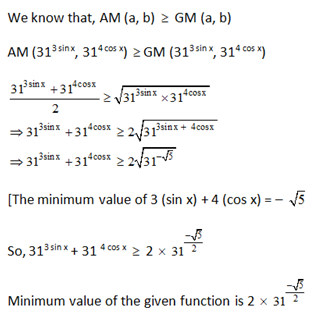11!! = 11×9×7×5×3×1 [X!! = X (X –2) (X – 4) …..  And so on]

= 111×32×71×51×31

Thus, the least perfect square which is divisible by 11!! Should be (111  32 71   51  31)  (111  71   51  31)

= 10395×1155 = 12006225.

120 = 23×31×51

Here are of such pairs

= 1 + (p + q + r) + 2 (pq + qr + pr) + 4pqr

(p = 3, q = 1, r = 1)

= 1 + 5 + 2 (3 + 1 + 3) + 4×3×1×1

= 6 + 14 + 12

= 32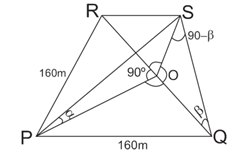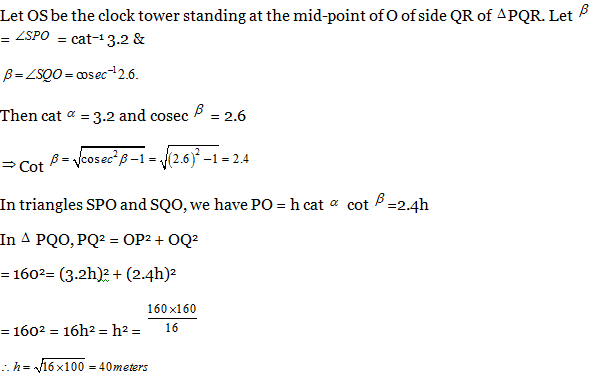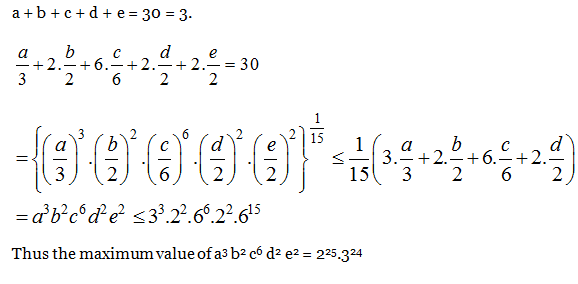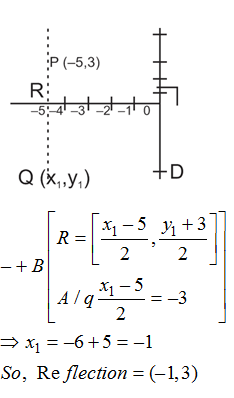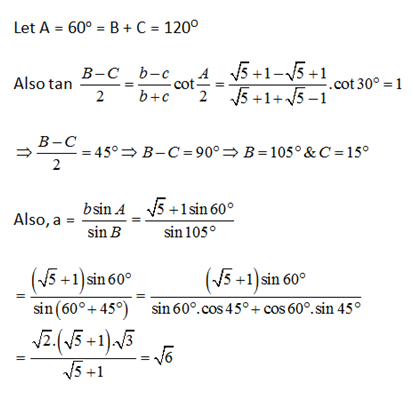### *******************

#### SSC CGL EXAMS 2019 |Quantitative Aptitude Practice Questions (Day-19)